# Optimization Methods Unconstrained optimization of an objective function

• Slides: 12Optimization Methods • Unconstrained optimization of an objective function F • Deterministic, gradient-based methods • Running a PDE: will cover later in course • Gradient-based (ascent/descent) methods • Stochastic methods • Simulated annealing • Theoretically but not practically interesting • Evolutionary (genetic) algorithms • Multiscale methods • Mean field annealing, graduated nonconvexity, etc. • Constrained optimization • Lagrange multipliers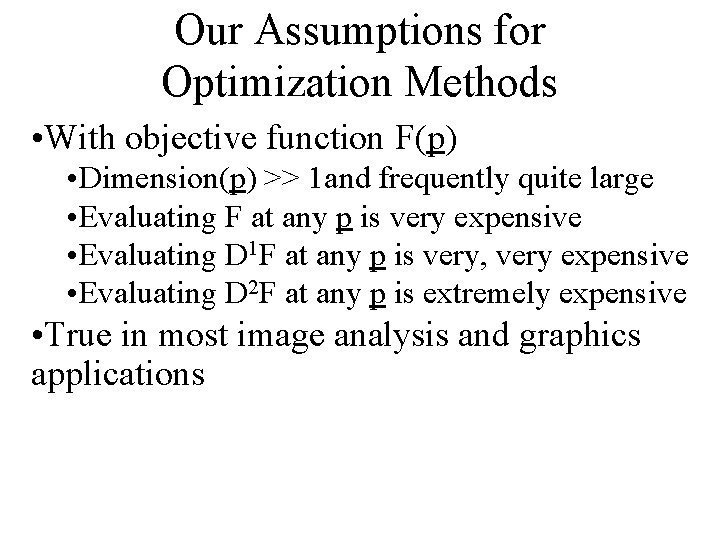Our Assumptions for Optimization Methods • With objective function F(p) • Dimension(p) >> 1 and frequently quite large • Evaluating F at any p is very expensive • Evaluating D 1 F at any p is very, very expensive • Evaluating D 2 F at any p is extremely expensive • True in most image analysis and graphics applications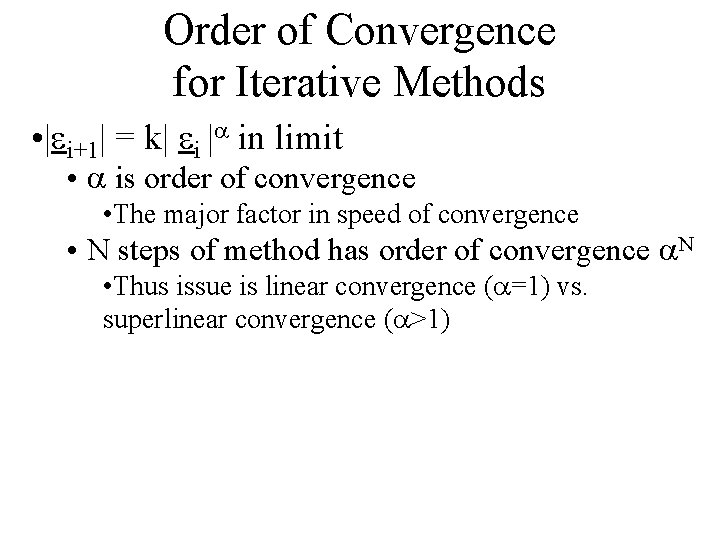Order of Convergence for Iterative Methods • |ei+1| = k| ei |a in limit • a is order of convergence • The major factor in speed of convergence • N steps of method has order of convergence a. N • Thus issue is linear convergence (a=1) vs. superlinear convergence (a>1)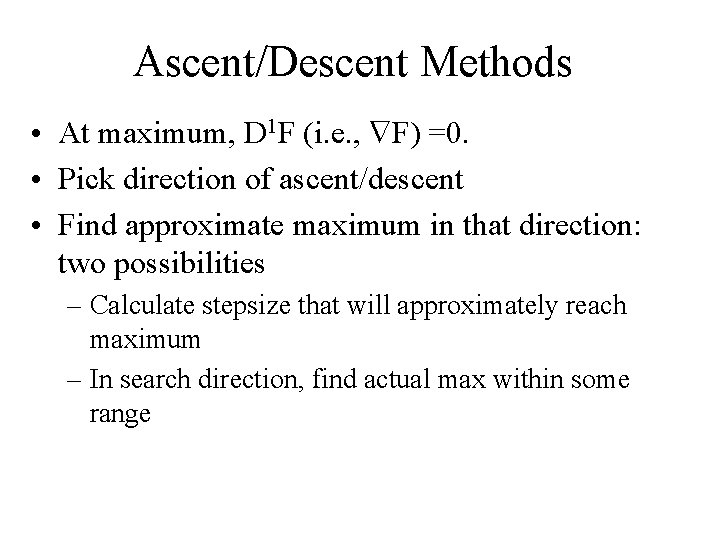Ascent/Descent Methods • At maximum, D 1 F (i. e. , F) =0. • Pick direction of ascent/descent • Find approximate maximum in that direction: two possibilities – Calculate stepsize that will approximately reach maximum – In search direction, find actual max within some range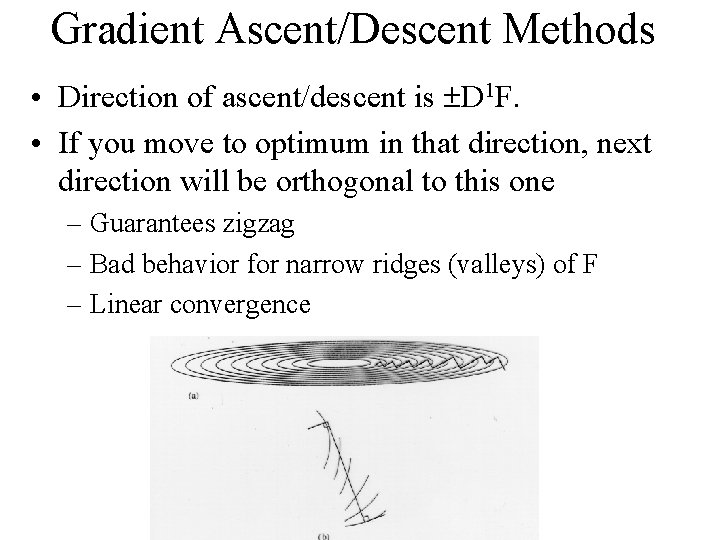Gradient Ascent/Descent Methods • Direction of ascent/descent is D 1 F. • If you move to optimum in that direction, next direction will be orthogonal to this one – Guarantees zigzag – Bad behavior for narrow ridges (valleys) of F – Linear convergence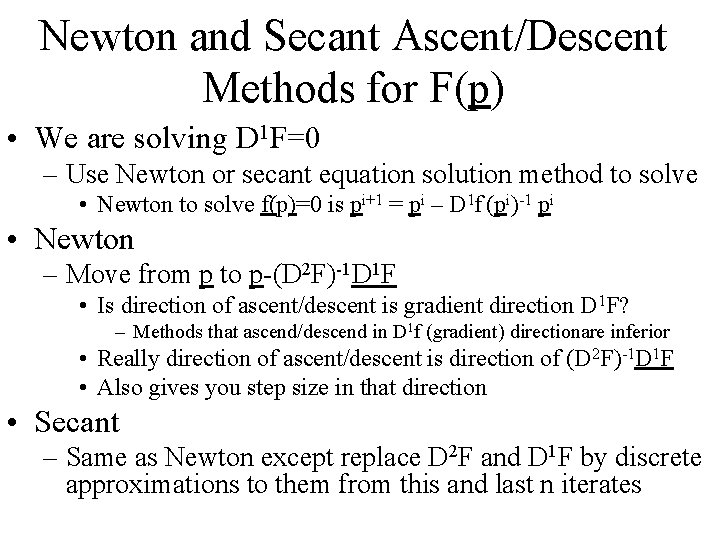Newton and Secant Ascent/Descent Methods for F(p) • We are solving D 1 F=0 – Use Newton or secant equation solution method to solve • Newton to solve f(p)=0 is pi+1 = pi – D 1 f (pi)-1 pi • Newton – Move from p to p-(D 2 F)-1 D 1 F • Is direction of ascent/descent is gradient direction D 1 F? – Methods that ascend/descend in D 1 f (gradient) directionare inferior • Really direction of ascent/descent is direction of (D 2 F)-1 D 1 F • Also gives you step size in that direction • Secant – Same as Newton except replace D 2 F and D 1 F by discrete approximations to them from this and last n iterates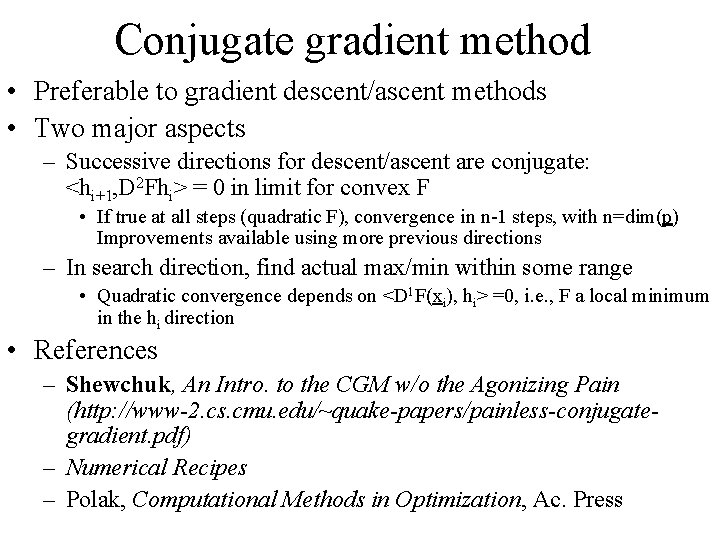Conjugate gradient method • Preferable to gradient descent/ascent methods • Two major aspects – Successive directions for descent/ascent are conjugate: <hi+1, D 2 Fhi> = 0 in limit for convex F • If true at all steps (quadratic F), convergence in n-1 steps, with n=dim(p) Improvements available using more previous directions – In search direction, find actual max/min within some range • Quadratic convergence depends on <D 1 F(xi), hi> =0, i. e. , F a local minimum in the hi direction • References – Shewchuk, An Intro. to the CGM w/o the Agonizing Pain (http: //www-2. cs. cmu. edu/~quake-papers/painless-conjugategradient. pdf) – Numerical Recipes – Polak, Computational Methods in Optimization, Ac. Press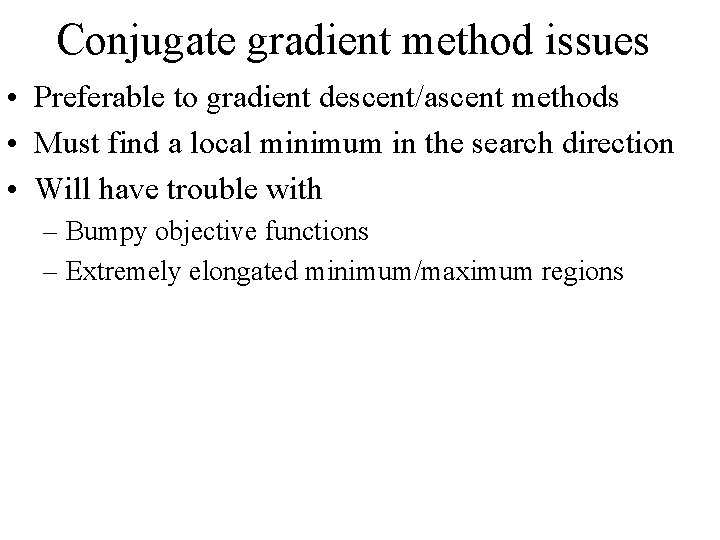Conjugate gradient method issues • Preferable to gradient descent/ascent methods • Must find a local minimum in the search direction • Will have trouble with – Bumpy objective functions – Extremely elongated minimum/maximum regions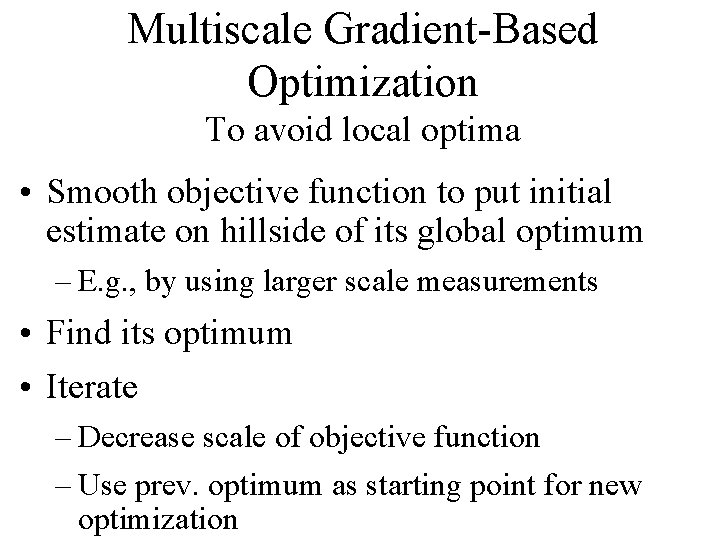Multiscale Gradient-Based Optimization To avoid local optima • Smooth objective function to put initial estimate on hillside of its global optimum – E. g. , by using larger scale measurements • Find its optimum • Iterate – Decrease scale of objective function – Use prev. optimum as starting point for new optimizationMultiscale Gradient-Based Optimization Example Methods • General methods – Graduated non-convexity • [Blake & Zisserman, 1987] – Mean field annealing • [Bilbro, Snyder, et al, 1992] • In image analysis – Vary degree of globality of geometric representation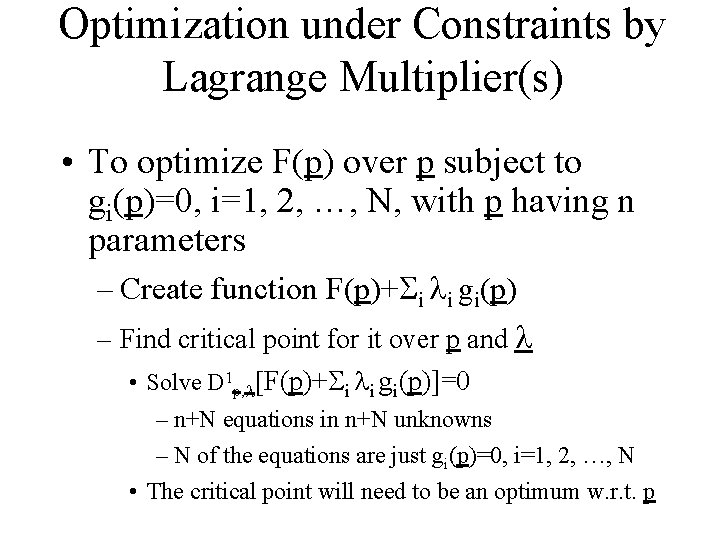Optimization under Constraints by Lagrange Multiplier(s) • To optimize F(p) over p subject to gi(p)=0, i=1, 2, …, N, with p having n parameters – Create function F(p)+ i li gi(p) – Find critical point for it over p and l • Solve D 1 p, l[F(p)+ i li gi(p)]=0 – n+N equations in n+N unknowns – N of the equations are just gi(p)=0, i=1, 2, …, N • The critical point will need to be an optimum w. r. t. pStochastic Methods • Needed when objective function is bumpy or many variables or hard to compute gradient of objective function